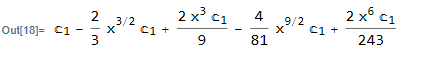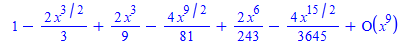# Question:Can maple solve an ode using asymptotic expansions methods?

## Question:Can maple solve an ode using asymptotic expansions methods?

Maple 2021

Can Maple solve an ode by asymptotic expansions methods? series methods (power series or Frobenius series) work only on ordinary expansion point or removable singularity point. For non removable singularity (essential), Maple's dsolve with series method can't solve these. Also, if the RHS of the ode is not analytic at the expansion point, series method do not work.

The asympt command only applies to algebraic expressions.

Does Maple have something similar to Mathematica's AsymptoticDSolveValue  ?

Here is an example. This is an ode with RHS not analytic (has no Taylor series) at x=0

```ode:=diff(y(x),x)+y(x)=1/x;
dsolve(ode,y(x),'series',x=0)```

No solution.  While in Mathematica

```ode = y'[x] + y[x] == 1/x
AsymptoticDSolveValue[ode, y[x], {x, 0, 6}]```Here is another example of ode where the coefficient of y(x) is not analytic at x=0

```ode:=diff(y(x),x)+sqrt(x)*y(x)=0;
dsolve(ode,y(x),'series',x=0)```

No solution. In Mathematica

```ode = y'[x] + Sqrt[x] y[x] == 0
AsymptoticDSolveValue[ode, y[x], {x, 0, 6}]```Does there exist a package in Maple that can do this? Will this functionality be added in Maple in the future if currently there is no support? ( I searched and could not find anything so far).

One possible workaround I found is to solve the ODE using normal methods (non-series), then apply the asympt command on the solution. But one needs to remove the constant of integration first, Here is the above example done this way

```ode:=diff(y(x),x)+sqrt(x)*y(x)=0;
sol:=dsolve(ode);
Order:=8;
sol:=eval(sol,_C1=1);
asympt(eval(rhs(sol),x=1/y),y);
eval(%,y=1/x)

```Mutliplying the above back by the constant _C1 gives same answer as Mathematica's.

Is the above how one is supposed to do it in Maple? It worked on the above simple example, but need to see if it will work for other examples. it will be better if this was more directly supported. i.e. if asympt will work directly on ode's (or a new command or a new option to dsolve similar to 'series' but call 'asympt').﻿